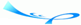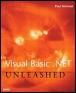# OperatorsTeam-FlyVisual Basic .NET Unleashed By Paul Kimmel Table of Contents Chapter 2.  Out with the Old, In with the New

Operators

To review, operators are the special symbols, such as +, /, Mod, and AndOr, that allow you to perform arithmetic, bitwise, comparison, concatenation, and logical operations in statements. Each operator takes a prescribed number and type of operands, or data.

Several new operators have been added to Visual Basic .NET. Some of these operators are designed to support bitwise logic and others have been defined to provide an abbreviated form of standard arithmetic. Several new assignment operators have been borrowed from C++. Look over Table 2.8 for a review of VB .NET operators.

##### Table 2.8. Visual Basic .NET Operators
Action Symbol Description
Binary Operands
Exponentiation ^ Written x^y; raises x to power y
Subtraction - x-y; performs subtraction and as an unary operator, negation
Multiplication * x*y; performs multiplication
Floating-point Division / x/y; floating-point division returns Double, except returns Single if both operands are Byte, Integer, or Single; returns Decimal if either operand is a Decimal
Integer Division \ x\y; integer division
Modulo Division Mod x Mod y; returns remainder of division of x by y
Addition + x + y; sum of x and y
Assignment = x = y; assigns value of y to x
Exponentiation Assignment ^= x ^= y; raises x to the power y and assigns the result to x
Multiplication Assignment *= x *= y; multiplication of x and y and assignment to x
Floating-point Division and Assignment /= x /= y; floating-point division of x by y and assignment to x
Integer Division and Assignment \= x \= y; integer division of x by y and assignment to x
Addition and Assignment += x += y; sum of x and y and assignment to x
Subtraction and Assignment -= x -= y; subtraction of y from x and assignment to x
Concatenation and Assignment &= x &= y, where x and y are strings; concatenation of x and y and assignment to x
Equality = x = y; tests for equality
Inequality <> x <> y; tests for inequality
Binary Operands
Less Than < x < y; tests for x less than y
Greater Than > x > y; tests for x greater than y
Less Than or Equal to <= x <= y; tests for x less than or equal to y
Greater Than Or Equal to >= x >= y; tests for x greater than or equal to y
Like Like string Like pattern ; compares string argument to pattern argument.
Is Is object1 is object2 ; tests to determine if object1 and object2 refer to the same object
Concatenation & string1 & any_expression ; converts right-hand-side argument to string and concatenates to left-hand-side argument
Concatenation + string1 + string2 ; concatenates two strings; may cause error if one of the left or right operands isn't a string
And And Bool1 And bool2 or x And y; logical And for Booleans and bitwise And for integrals
Or Or Applies to bool1 Or bool2 and x Or y; logical Or on Booleans and bitwise Or on integrals
Xor Xor Bool1 Xor bool2 or x Xor y; logical exclusive-Or for Booleans and bitwise exclusive Oring of integrals
AndAlso AndAlso Short-circuited And operation
OrElse OrElse Short-circuited Or operation
Unary Operands
GetType GetType GetType( any ); returns runtime type of argument
Not Unary Not bool or Not x; logical negation for Booleans and bitwise negation for integrals

Many of these operators you've seen before. We won't rehash those. A few others are new and AddressOf has taken on new importance. Let's take a moment to cover new or changed operators.

All operators of the form token = perform two operations. For example, I += 5 is expanded to I = I + 5. These combined operators have been around in C++ for about 12 years or so.

AddressOf has taken on new importance in VB .NET. In VB6 you could use the AddressOf operator to pass the address of a procedure to APIs that needed a callback procedure. (A callback is simply a procedure invoked by its address.) In VB .NET you can create your own callbacks and use them in your program. Callbacks are supported directly with the delegate idiom. Read Chapter 9, "Understanding Delegates," for more on this advanced topic.Team-FlyTopVisual BasicR. NET Unleashed
ISBN: N/A
EAN: N/A
Year: 2001
Pages: 222